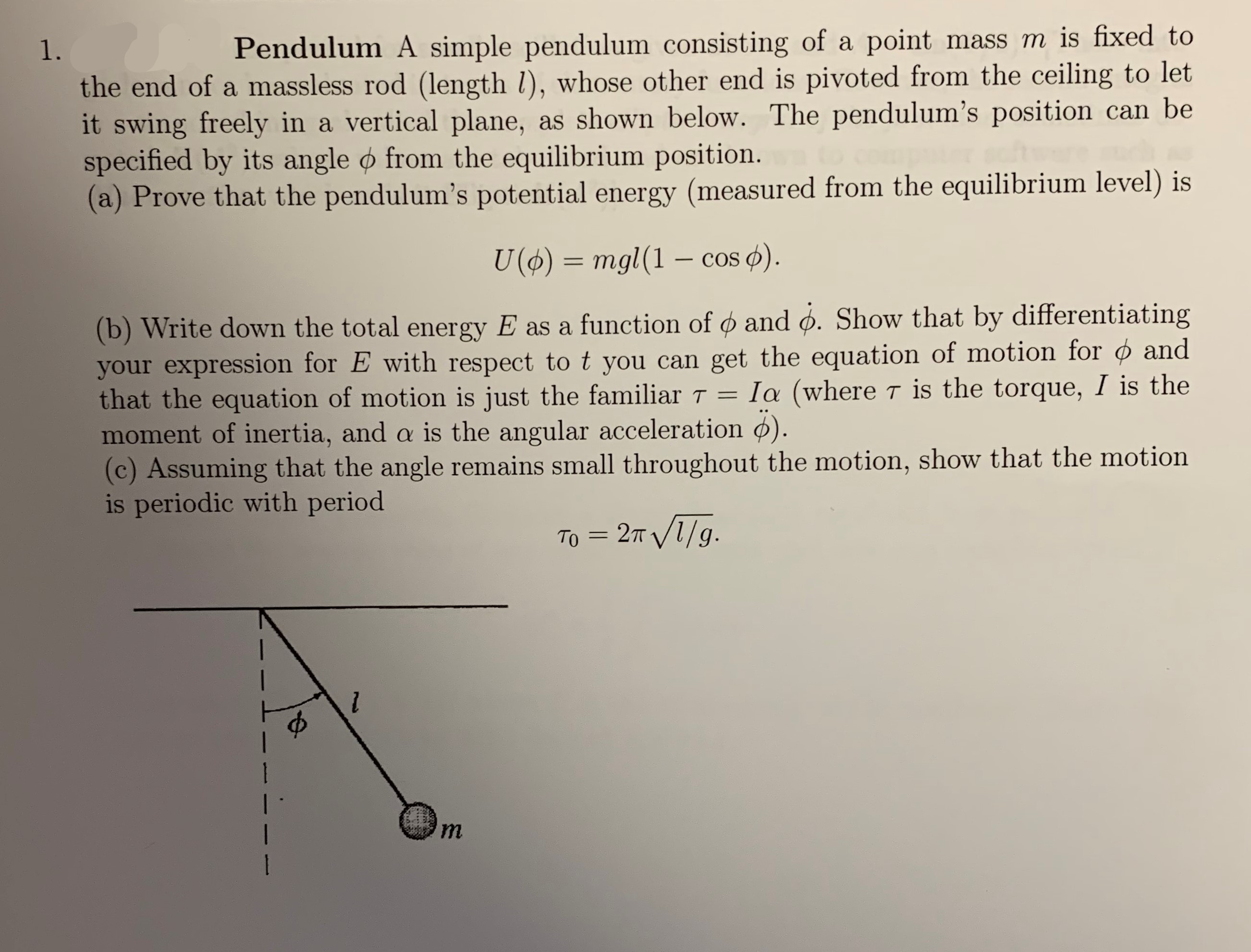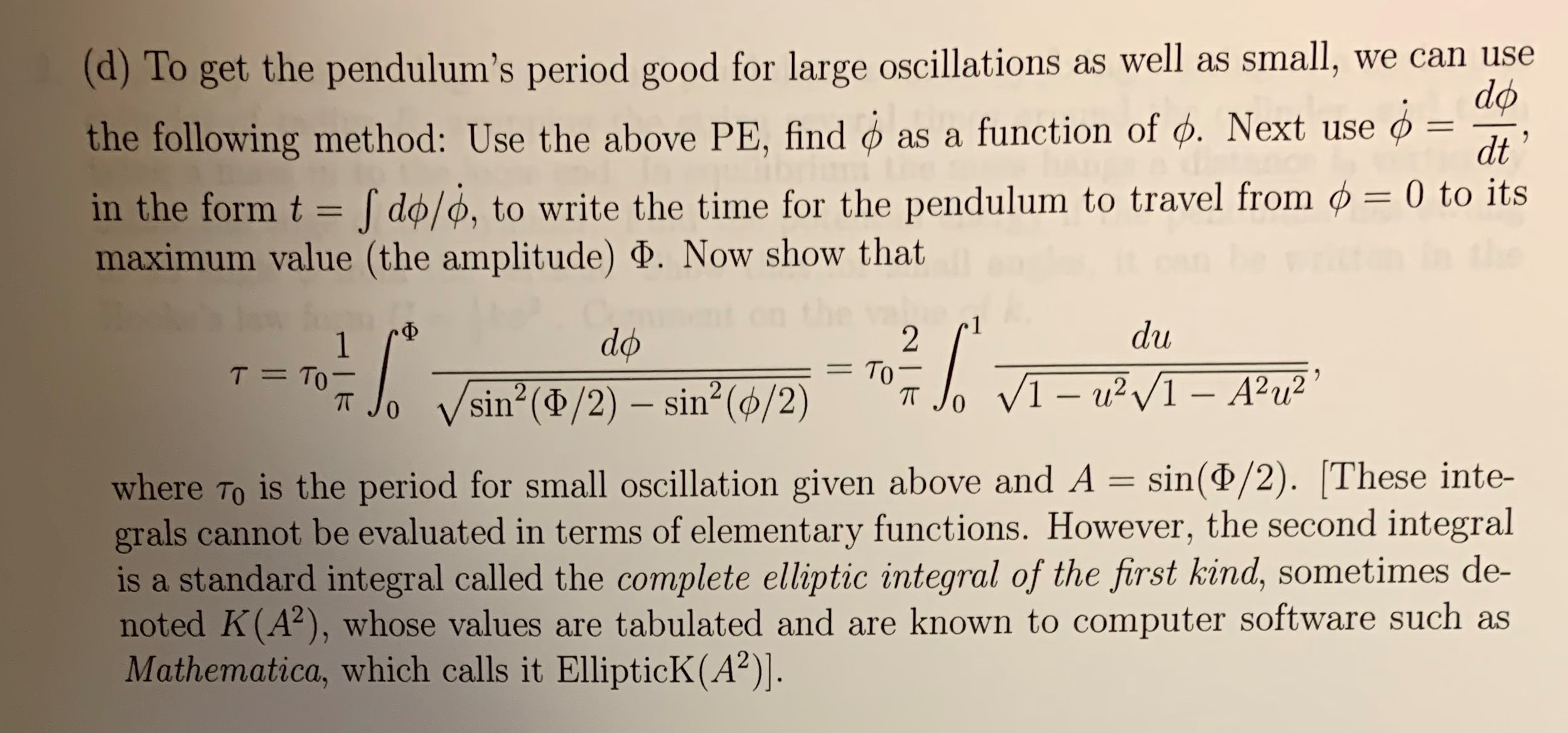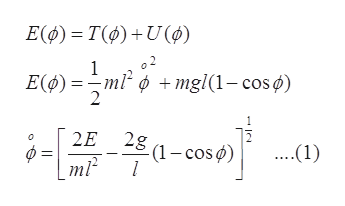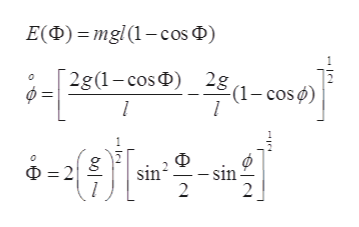mass m is fixed toPendulum A simple pendulum consisting of a point1.the end of a massless rod (length /), whose other end is pivoted from the ceiling to letit swing freely in a vertical plane,specified by its angle o from the equilibrium position.(a) Prove that the pendulum's potential energy (measured from the equilibrium level) isas shown below. The pendulum's position can beU ()mgl (1 - cos )(b) Write down the total energy E as a function of and o. Show that by differentiatingyour expression for E with respect to t you can get the equation of motion for ¢ andthat the equation of motion is just the familiar Tmoment of inertia, and a is the angular acceleration ).(c) Assuming that the angle remains small throughout the motion, show that the motionis periodic with periodIa (where T is the torque, I is the- 2т V1/9.Tоm1 (d)To get the pendulum's period good for large oscillations as well as small,the following method: Use the above PE, findwe can usedoas a function of d. Next use11dtin the form tdo/o, to write the time for the pendulum to travel from ø = 0 to itsmaximum value (the amplitude) . Now show thatdu-Фdo2То -T TO1VI-u2I-A2u2sin (d/2) sin2 (ø/2)0is the period for small oscillation given above and A = sin(P/2). These inte-where Tograls cannot be evaluated in terms of elementary functions. However, the second integralis a standard integral called the complete elliptic integral of the first kind, sometimes de-noted K(A2), whose values are tabulated and are known to computer software such asMathematica, which calls it EllipticK (A2)].

Question

can you do part d pleasehelp_outlineImage Transcriptionclosemass m is fixed to Pendulum A simple pendulum consisting of a point 1. the end of a massless rod (length /), whose other end is pivoted from the ceiling to let it swing freely in a vertical plane, specified by its angle o from the equilibrium position. (a) Prove that the pendulum's potential energy (measured from the equilibrium level) is as shown below. The pendulum's position can be U ()mgl (1 - cos ) (b) Write down the total energy E as a function of and o. Show that by differentiating your expression for E with respect to t you can get the equation of motion for ¢ and that the equation of motion is just the familiar T moment of inertia, and a is the angular acceleration ). (c) Assuming that the angle remains small throughout the motion, show that the motion is periodic with period Ia (where T is the torque, I is the - 2т V1/9. Tо m 1 fullscreenhelp_outlineImage Transcriptionclose(d)To get the pendulum's period good for large oscillations as well as small, the following method: Use the above PE, find we can use do as a function of d. Next use 11 dt in the form t do/o, to write the time for the pendulum to travel from ø = 0 to its maximum value (the amplitude) . Now show that du -Ф do 2 То - T TO 1 VI-u2I-A2u2 sin (d/2) sin2 (ø/2) 0 is the period for small oscillation given above and A = sin(P/2). These inte- where To grals cannot be evaluated in terms of elementary functions. However, the second integral is a standard integral called the complete elliptic integral of the first kind, sometimes de- noted K(A2), whose values are tabulated and are known to computer software such as Mathematica, which calls it EllipticK (A2)]. fullscreen
Step 1

Potential energy of simple pendulum is given by:

Step 2

Total energy of the pendulum E(φ) with Kinetic energy T(φ) and potential energy U(φ) at time t is:help_outlineImage TranscriptioncloseΕφ)-Τφ)+ U() ο2 1 Ε(φ)-ml? φ +mgl(1- cos φ) 2 4-ΗΗ-ωo). 2Ε 2g .1) (1-cos φ) ml2 fullscreen
Step 3

At the extreme point φ = 0 and φ = Φ we get equation ...help_outlineImage TranscriptioncloseE(D)mgl(1-cos D) 2g(1-cosD 281-cos) l 마을 빠를을 D2 sin - sin 2 L | fullscreen

Want to see the full answer?

See Solution

Want to see this answer and more?

Our solutions are written by experts, many with advanced degrees, and available 24/7

See Solution
Tagged in

Science